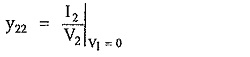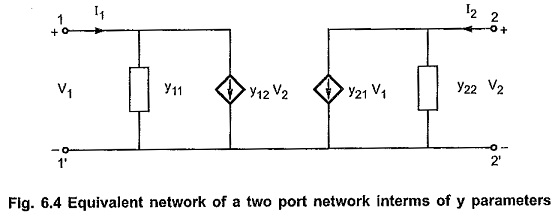## y Parameters of Two Port Network:

y Parameters of Two Port Network are also called admittance parameters. These are obtained by expressing currents at two ports in terms of voltages at two ports. Thus, voltages V1 and V2 are independent variables, while I1 and I2 are dependent variables. Thus, we have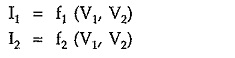In equation form, above relations can be written as,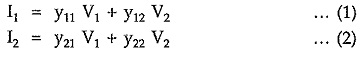In matrix form, above equations can be written as,The individual y-parameters are defined as follows.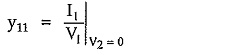This is called driving point input admittance.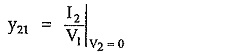This is called forward transfer admittance.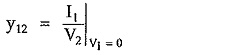This is called reverse transfer admittance